104095 人已学习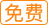开篇词 | 从今天起，重新理解前端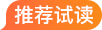15
15
1.0x
00:00/00:00

# CSS渲染：CSS是如何绘制颜色的？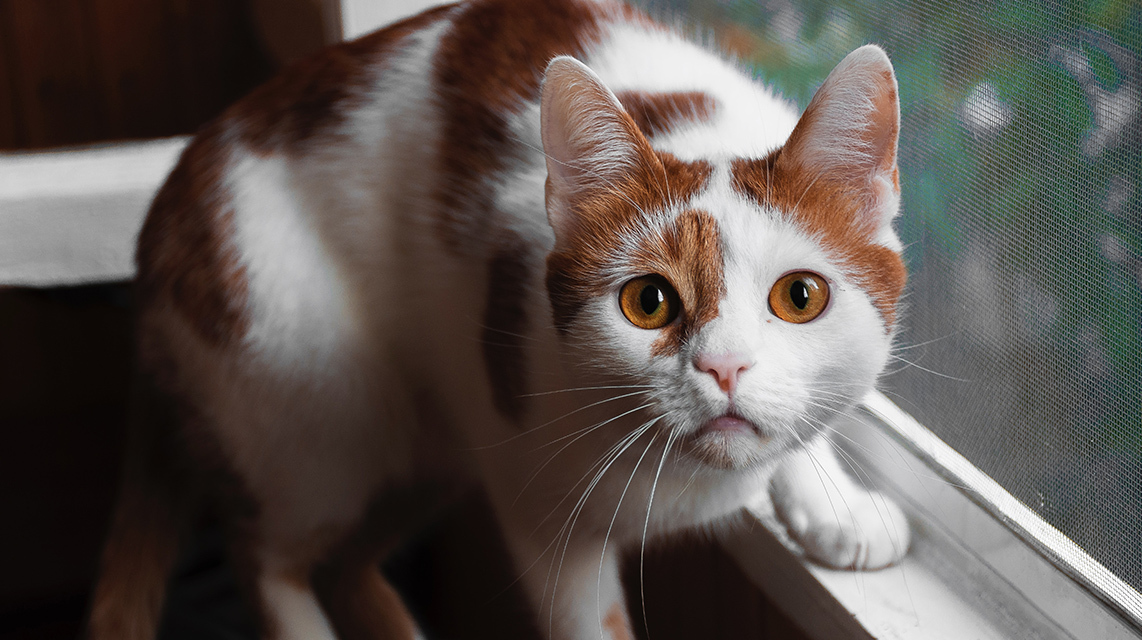## 颜色的原理

### RGB 颜色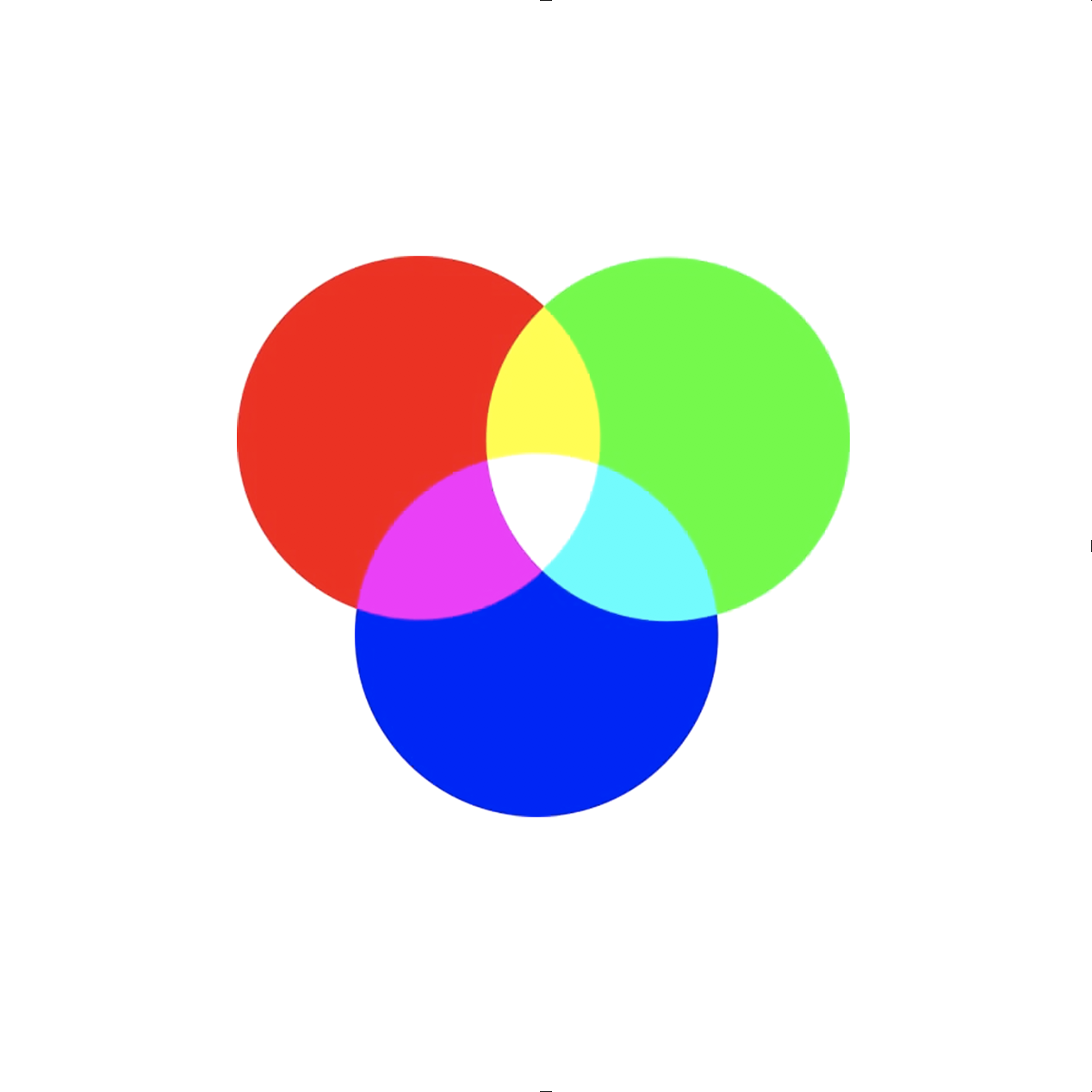0/2000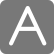荧光笔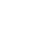直线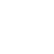曲线

AI
• 深入了解
• 翻译
• 英语
• 中文简体
• 中文繁体
• 法语
• 德语
• 日语
• 韩语
• 俄语
• 西班牙语
• 阿拉伯语
• 解释
• 总结

《重学前端》## 全部留言(14)

• 最新
• 精选
•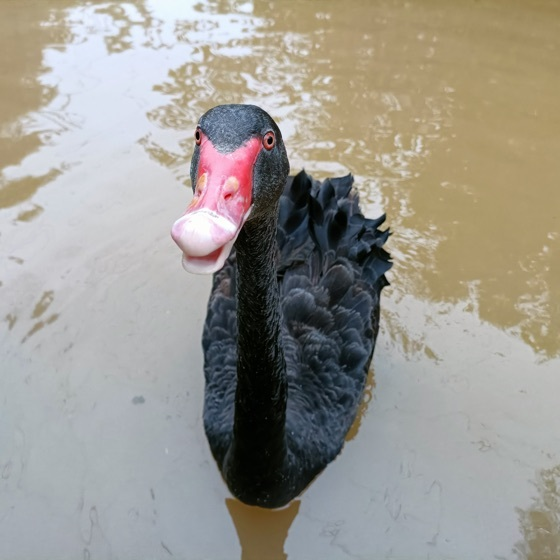阿成
怎么说呢，要想完美的转换... 好难... 仅靠单像素颜色来识别出鹦鹉的轮廓还是不太可行... 也许把周围像素的颜色考虑进去是个办法... 不过这图挺大的... <!DOCTYPE html> <html lang="en"> <head> <meta charset="UTF-8"> <title>Document</title> <style type="text/css"> .bird { width: 400px; height: calc(1440 * 400 / 1920 * 1px); } canvas.bird { background: #ccc; } </style> </head> <body> <img id="img" class="bird" src="./bird.jpg"> <canvas id="canvas" width="1920" height="1440" class="bird"></canvas> <script type="text/javascript"> let canvas = document.getElementById('canvas') let ctx = canvas.getContext('2d') let img = document.getElementById('img') img.addEventListener('load', () => { ctx.drawImage(img, 0, 0) let imageData = ctx.getImageData(0, 0, canvas.width, canvas.height) let data = imageData.data for (let i = 0; i < data.length; i += 4) { if (isBird(data, i, canvas.width, canvas.height)) { ;[data[i], data[i + 1]] = [data[i + 1] * 1.2, data[i]] } } ctx.putImageData(imageData, 0, 0) }) function isBird (data, i, width, height) { let r = data[i] let g = data[i + 1] let b = data[i + 2] let [h, s, l] = rgb2hsl(r, g, b) return h < 200 && h > 80 && s > 0.23 && l < 0.84 } function rgb2hsl (r, g, b) { let r1 = r / 255 let g1 = g / 255 let b1 = b / 255 let min = Math.min(r1, g1, b1) let max = Math.max(r1, g1, b1) let l = (min + max) / 2 let s let h if (l < 0.5) { s = (max - min) / (max + min) } else { s = (max - min) / (2 - max - min) } if (max === r1) { h = (r1 - b1) / (max - min) } else if (max === g1) { h = 2 + (b1 - r1) / (max - min) } else if (max === b1) { h = 4 + (r1 - g1) / (max - min) } h *= 60 while (h < 0) { h += 360 } return [h, s, l] } </script> </body> </html>
7
35
•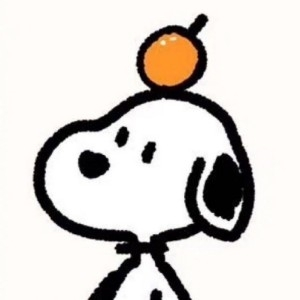Peter
一步到位：filter: hue-rotate(240deg); 根据HSL色环，绿色在120deg的位置，要变成红色，把色相顺时针旋转240deg或者逆时针120deg即可。
2
24
•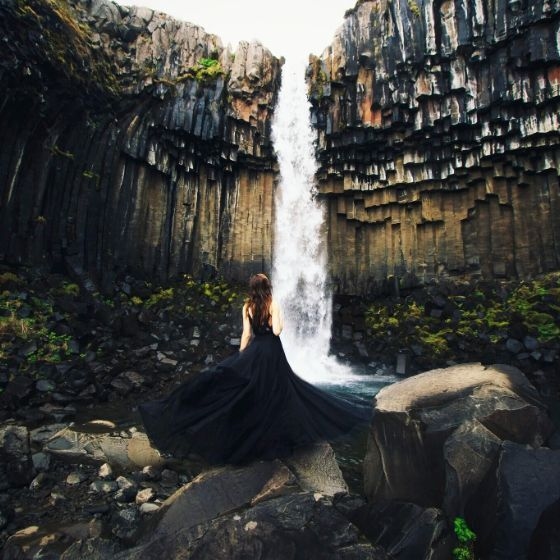GGFGG
CMYK，为什么有K，一方面是成本，另一方面是因为自然界的CMY不能合成纯黑的颜色，所以需要纯黑
8
•sugar
我来给个答案吧，乍一看 感觉需要用到很多cv领域的技术，模式识别判定轮廓，然后根据色值不同进行greenToRed转译。后来想了一下，这明明是前端的课程嘛，按cv的解决方案，难道还要把opencv编译到wasm里？转念一想，其实css滤镜就能做这事儿，试了试 几行css代码能做的事 在opencv要引一大堆库 改一大堆参数了
2
7
•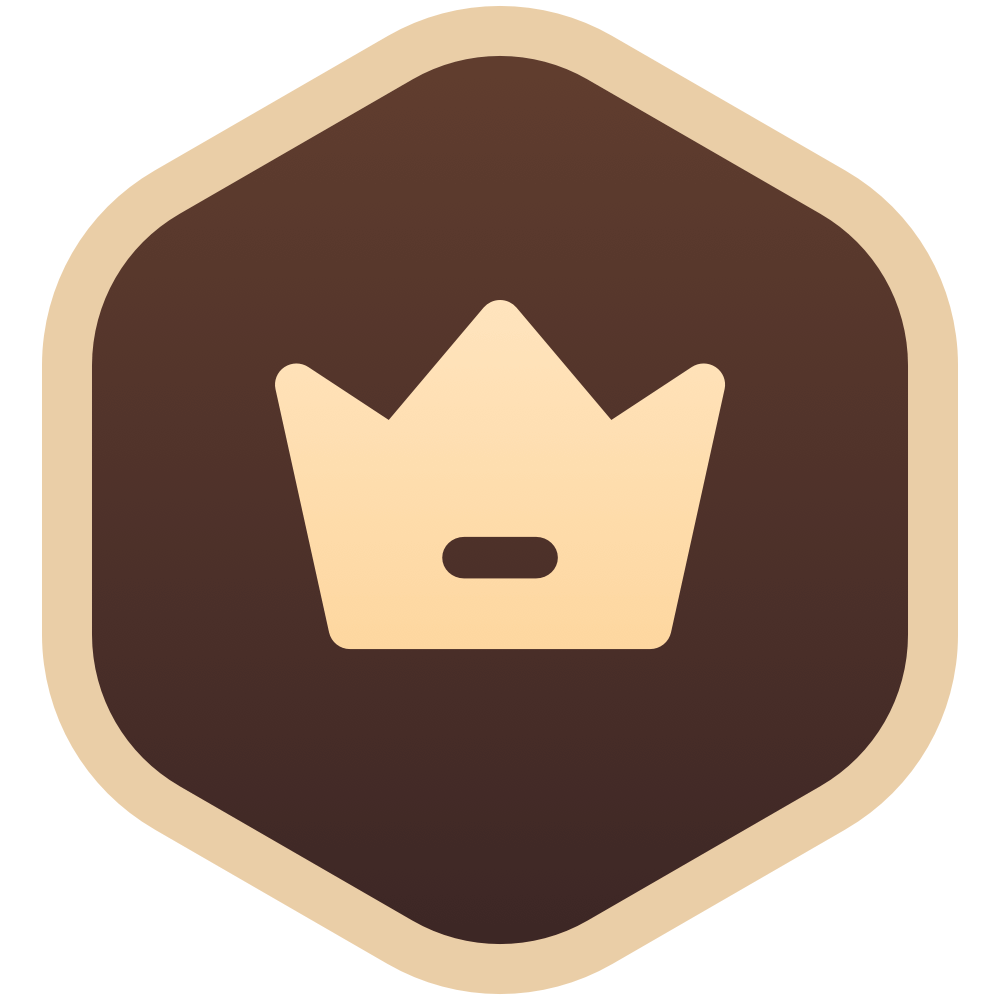KL宇<!DOCTYPE html> <html> <head> <title>鹦鹉变成红色</title> <script> function loadImg() { let img = new Image img.src = "yingwu.jpg" img.onload = function () { drawCanvas(img) } } function drawCanvas(img) { let canvas = document.getElementById('canvas') canvas.width = img.width canvas.height = img.height let context = canvas.getContext('2d') context.drawImage(img, 0, 0); //context.clearRect(200,432, 1110, 670); let sectionImg = context.getImageData(200, 432, 1110, 75 0); let imgData = sectionImg.data; for(let i =1; i < imgData.length;i += 4) { if (imgData[i-1] < imgData[i]) { let temp = imgData[i-1] imgData[i - 1] = imgData[i] imgData[i] = temp } } context.putImageData(sectionImg, 200, 432); // 复制代码 } document.addEventListener('DOMContentLoaded', function(){ loadImg() }) </script> </head> <body> <canvas id="canvas"></canvas> </body> </html>
2
•一路向北
4
2
•猫总
原本实现控制RGB范围来手动抠图，不过在使用的时候发现并不直观，调整起来很随缘，回看了一遍课程才发现重点是HSL调色，改进之后还是能比较精准（主要是直观）的把鹦鹉给单独替换颜色了
1
•Geek_0bb537
winter老师给我讲一下那个presentational attributes 看不懂
1
•Izayoizuki
HSL感觉还是绘画游戏原画之类用得多，编程领域反而挺少，无论h5游戏还是客户端游戏理解一般都是rgb/rgba
1
•Aaaaaaaaaaayou
canvas可以得到每个像素的rgb分量，是不是把蓝色和红色的值换一下就可以了？
1